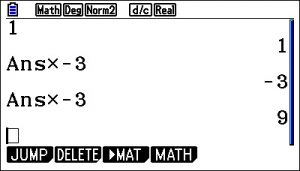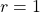# Geometric Sequences

A geometric sequence is an ordered set, usually of numbers, such that there is a common ratio between terms.

Here is an example:Here is another example:Notice that in the first example, the common ratio is 3. In the second example, the common ratio is -3.

You can create a sequence such as this on a scientific or graphical display calculator, by typing in 1, enter, multiply (-3) enter, enter, enter…# Fill in the Gaps

The following applets generate geometric sequences. Using your own technique figure out the numbers in the gaps.

# Formula

To find any term in a geometric sequence given the first term, and the common ratio, we can use the formula.

Try this formula out with the applet ‘find a particular term’ above.

# 3 kinds of common ratio

1. When the ratio is bigger than 1, the sequences gets bigger and bigger. We say it diverges.
2. When the ratio is negative, the sequence oscillates between positive and negative values.
3. When the ratio is between -1 and 1 (but not zero), the sequence diminishes. We say it converges to zero.

In the following applet, the first term is 20. Try out:

• common ratio = 1.2 (ratio is positive, bigger than 1).
• common ratio = -1.2 (ratio is negative)
• common ratio = 0.5 (ratio is positive, less than 1)

Notice the various patterns made by the points.

What about,,?